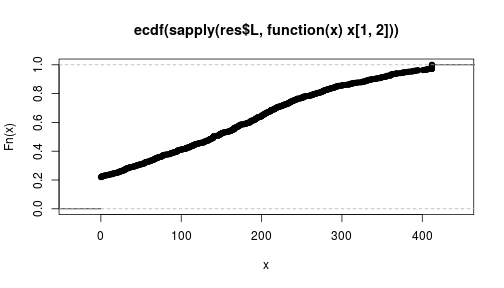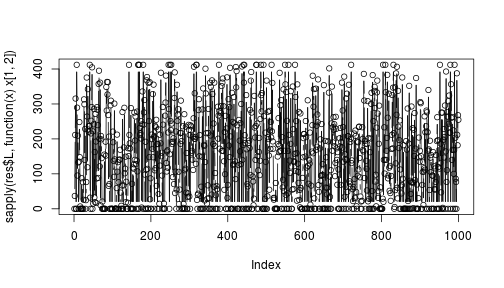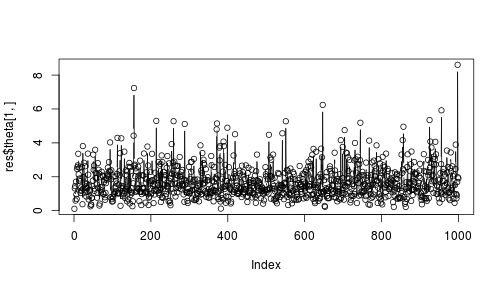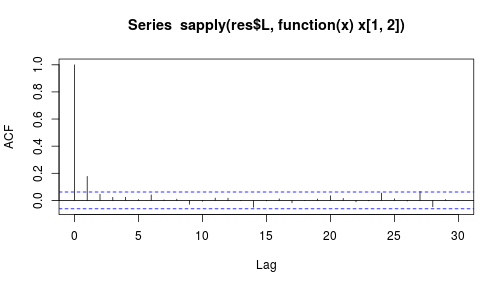# An example using the fitness model

This document describe a toy example for the use of the package systemicrisk.

``````library(systemicrisk)
``````

Suppose we observe the following vector of total liabilities and todal assets.

``````l <- c(714,745,246, 51,847)
a <- c(872, 412, 65, 46,1208)
``````

The following sets up a model for 5 banks:

``````mod <- Model.additivelink.exponential.fitness(n=5,alpha=-2.5,beta=0.3,gamma=1.0,
lambdaprior=Model.fitness.genlambdaparprior(ratescale=500))
``````

Choosing thinning to ensure sample is equivalent to number of

``````thin <- choosethin(l=l,a=a,model=mod,silent=TRUE)
``````
``````## Warning in findFeasibleMatrix_targetmean(l, a, p = u\$p, targetmean =
## mean(genL(model)\$L > : Desired mean degree is less than minimal degree that
## is necessary.

## Warning in findFeasibleMatrix_targetmean(l, a, p = u\$p, targetmean =
## mean(genL(model)\$L > : Desired mean degree is less than minimal degree that
## is necessary.
``````
``````thin
``````
``````##  60
``````

Running the sampler to produce 1000 samples.

``````res <- sample_HierarchicalModel(l=l,a=a,model=mod,nsamples=1e3,thin=thin,silent=TRUE)
``````
``````## Warning in findFeasibleMatrix_targetmean(l, a, p = u\$p, targetmean =
## mean(genL(model)\$L > : Desired mean degree is less than minimal degree that
## is necessary.
``````

Some examples of the matrics generated are below.

``````res\$L[]
``````
``````##      [,1] [,2] [,3] [,4] [,5]
## [1,]    0    0    0    0  714
## [2,]  497    0    0    0  248
## [3,]    0    0    0    0  246
## [4,]    0   51    0    0    0
## [5,]  375  361   65   46    0
``````
``````res\$L[]
``````
``````##          [,1]       [,2]      [,3]     [,4]      [,5]
## [1,]   0.0000  36.763750  3.163915 26.61477 647.45756
## [2,] 169.2638   0.000000 61.836085  0.00000 513.90011
## [3,]   0.0000 226.614770  0.000000 19.38523   0.00000
## [4,]   0.0000   4.357677  0.000000  0.00000  46.64232
## [5,] 702.7362 144.263803  0.000000  0.00000   0.00000
``````

The sampler produces samples from the conditional distribution of matrix and parameter values given the observed data. To see the posterior distribution of the liabilities of Bank 1 towards Bank 2:

``````plot(ecdf(sapply(res\$L,function(x)x[1,2])))
``````## Diagnostic of the R-output

All the caveats of MCMC algorithms apply. In particular the samples are dependent.

Some automatic diagnostic can be generated via the function diagnose.

``````diagnose(res)
``````
``````## Analysis does not consider 5 entries of matrix
## that are deterministic (diagonal elements, row/column sum=0 or forced result).
## All remaining elements of the liabilities matrix have moved during sample run.
## ESS in matrix:
##    Min. 1st Qu.  Median    Mean 3rd Qu.    Max.
##   600.8   693.3  1000.0   880.0  1000.0  1000.0
## ESS in theta:
##    Min. 1st Qu.  Median    Mean 3rd Qu.    Max.
##   447.2   717.7   751.6   766.3   864.9  1000.0
``````

Trace plots of individual liabilities also shoud show rapid mixing - as seems to be the case for the liabilities of Bank 1 towards Bank 2.

``````plot(sapply(res\$L,function(x)x[1,2]),type="b")
``````Trace plot of the fitness of bank 1.

``````plot(res\$theta[1,],type="b")
``````Also, the autocorrelation function should decline quickly. Again, considering the liabilities between bank 1 and bank 2:

``````acf(sapply(res\$L,function(x)x[1,2]))
``````In this case it decays quickly below the white-noise threshold (the horizontal dashed lines).